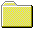Back to Tape Recording Page

# HD3 v level at 315 Hz for 104 different cassette tapes

## Type I (position "Normal") - 41 cassettes Type II (position "Chrome") - 46 cassettes Type IV (position "Metal") - 17 cassettes

Measurement Setup:

Nakamichi BX-300E cassette deck
PC with RME Digi 96/8 PAD sound card
HP 3478 RMS multimeter
AudioTester Software.

Level 250mV on the graphs is equivalent to 250 nWb/m (set by BASF reference tape).
Frequency is 315 Hz, measured 3rd harmonic only

Here is an approximate scale reference in mV v dB for this setup:

700 mV = +13 dB VU
600 mV = +11.5 dB VU
500 mV = +10 dB VU
400 mV = +8 dB VU
300 mV = +5.5 dB VU
250 mV = +4 dB VU = 250 nWb/m , DIN Reference Level
215 mV = +2.7 dB VU = "Dolby Level"
160 mV = 0VU
80 mV = -6 dB VU
40 mV = -12 dB VU
20 mV = -18 dB VU

NOTES:
on the X-axis shown the relative measured RMS output level from the tape in mV
on the Y-axis shown the 3rd harmonic in dB

Here is an approximate scale reference of HD3 level in dB v HD3 level in %:

-20 dB = 10 %
-30 dB = 3% , at this distortion level the Maximum Output Level (MOL) is usually measured
-40 dB = 1%
-50 dB = 0.3%
-60 dB = 0.1%
-70 dB = 0.03%TypeITypeIITypeIV